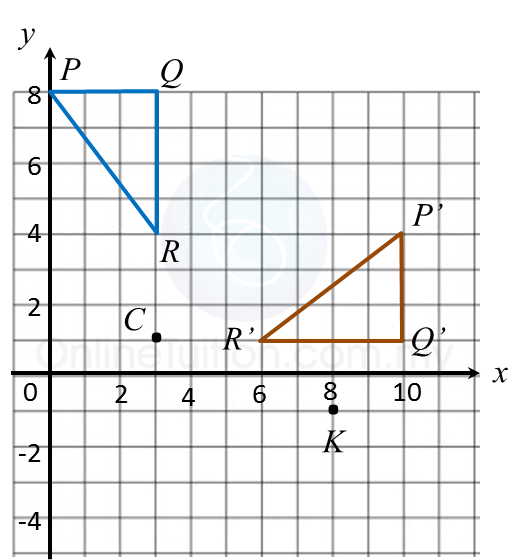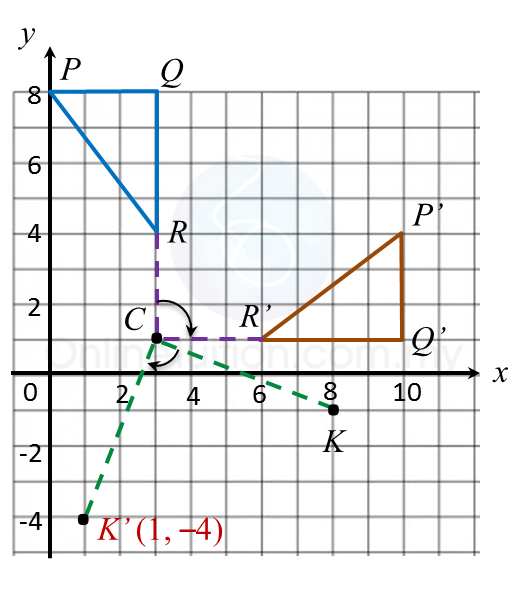# 11.2.1 Transformations (I), PT3 Focus Practice

11.2.1 Transformations (I), PT3 Focus Practice

Question 1:
Diagram below in the answer space shows object drawn on a grid of equal squares with sides of 1 unit.
On the diagram, draw the image of object under the translation $\left(\begin{array}{l}-6\\ \text{}3\end{array}\right).$

:Solution
: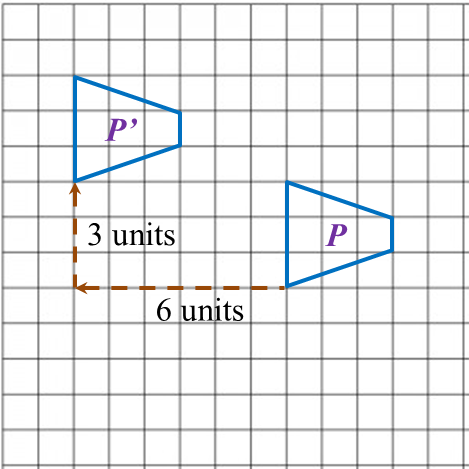Question 2:
Describe the translation which maps point P onto point P’.Solution
: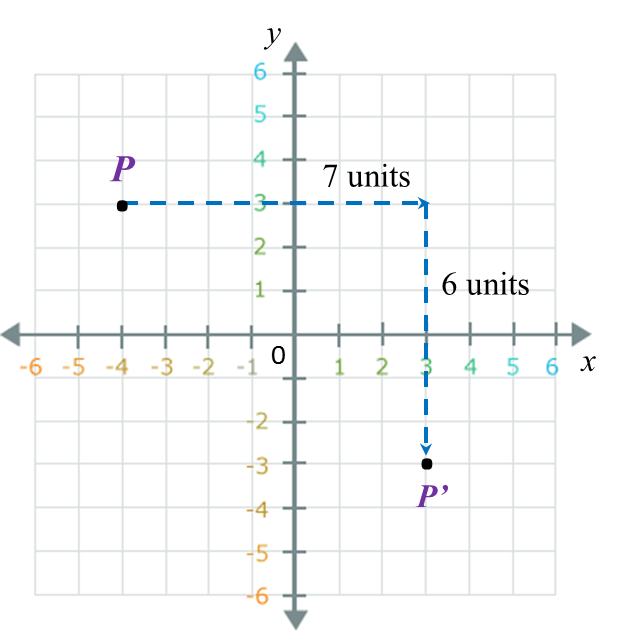The translation is $\left(\begin{array}{l}\text{}7\\ -6\end{array}\right).$

Question 3:
Diagram below in the answer space shows quadrilateral PQRS. R’S’ is the image of RS under a reflection in the straight line AB.
On diagram in the answer space, complete the image of quadrilateral PQRS.

: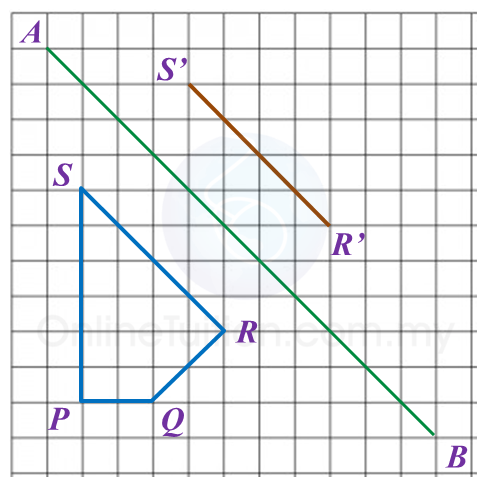Solution:Question 4:
Diagram in the answer space, shows two polygons, M and M’, drawn on a grid of equal squares with sides of 1 unit. M’ is the image of M under a reflection.
(a) Draw the axis of reflection.
(b) Mark the image of P under the same reflection.
(c) Draw the image of M under reflection in the x-axis.

:Solution:Question 5:
On diagram in the answer space, triangle P’Q’R’ is the image of triangle PQR under a rotation about centre C.
(a) State the angle and direction of the rotation.
(b) K’is the image of point K under the same rotation.
Mark and state the coordinates of K’.